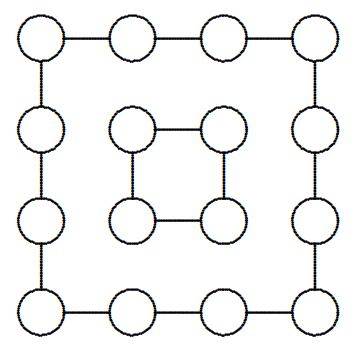# Placing Numbers in a Square 2

Logic Level 5Each of the integers from 1 to 16 is to be placed once each in one of the circles in the figure so that the integers along each of the four outer sides of the large figure and the four integers that make up the inner figure have identical sums. What is the largest sum, if any, that can be obtained?

×